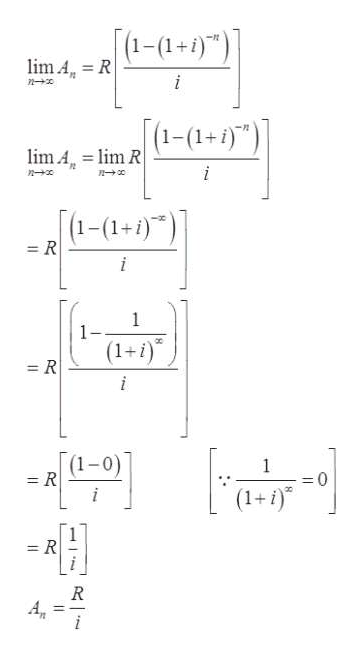# If an annuity makes an infinite series of equal payments at the end of the interest periods, it is called a perpetuity. If a lump sum investment of An is needed to result in n periodic payments of R when the interest rate per period is i, thenAn = R[(1-(1+i)^-n)/(i)](a) Evaluatelim n→∞ Anto find a formula for the lump sum payment for a perpetuity.  (b) Find the lump sum investment needed to make payments of \$180 per month in perpetuity if interest is 6%, compounded monthly. (Round your answer to the nearest cent.)\$

Question
61 views

If an annuity makes an infinite series of equal payments at the end of the interest periods, it is called a perpetuity. If a lump sum investment of An is needed to result in n periodic payments of R when the interest rate per period is i, then

An = R[(1-(1+i)^-n)/(i)]

(a) Evaluate
lim n→∞ An
to find a formula for the lump sum payment for a perpetuity.

(b) Find the lump sum investment needed to make payments of \$180 per month in perpetuity if interest is 6%, compounded monthly. (Round your answer to the nearest cent.)
\$
check_circle

Step 1

Given:

Step 2

(a). Find the limithelp_outlineImage Transcriptionclose[1-(1+)") lim AR i (1-(1+i)") lim A, lim R i 1-(1-)") = R 1 1- (1+) - R "संव (1-0) - R 1 - R fullscreen
Step 3

(b). Finding the lump investment needed to make payments of \$180 pe...

### Want to see the full answer?

See Solution

#### Want to see this answer and more?

Solutions are written by subject experts who are available 24/7. Questions are typically answered within 1 hour.*

See Solution
*Response times may vary by subject and question.
Tagged in

### Calculus